• ## lingo求解线性规划

千次阅读 2020-07-11 10:28:31
注：默认情况下，lingo规定变量是非负的，也是说下界为0，上界为正无穷。 二、模型以及对应程序 模型： lingo程序 Model: Max=72*x1+64*x2; x1+x2<50; 12*x1+8*x2<480; 3*x1<100; end ...
一、常用的变量界定
@bin(x)：限制x为0或1；
@bnd(L,x,U)：限制L  x  U;
@free(x)：取消对变量x的默认下界为0的限制，x可以为任意实数;
@gin(x)：限制x为整数.
注：默认情况下，lingo规定变量是非负的，也是说下界为0，上界为正无穷。
二、模型以及对应程序
模型：

lingo程序
Model:
Max=72*x1+64*x2;
x1+x2<50;
12*x1+8*x2<480;
3*x1<100;
end

展开全文• 2、MATLAB求解 2.1 代码 c=[2;3;1]; a=[1 4 2;3 2 0]; b=[8;6]; [x,y]=linprog(c,-a,-b,[],[],zeros(3,1)) 2.2 结果 Optimization terminated. x = 0.8066 1.7900 0.0166 y = 7.0000 3、l...
1、例子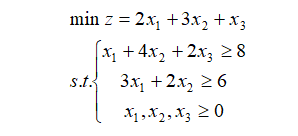2、MATLAB求解
2.1 代码
c=[2;3;1];
a=[1 4 2;3 2 0];
b=[8;6];
[x,y]=linprog(c,-a,-b,[],[],zeros(3,1))
2.2 结果
Optimization terminated.

x =

0.8066
1.7900
0.0166
y =

7.0000
3、lingo求解
3.1 代码
min=2*x1+3*x2+x3;
x1+4*x2+2*x3>=8;
3*x1+2*x2>=6;
x1>=0;
x2>=0;
x3>=0;
3.2 结果
 Global optimal solution found.
Objective value:                              7.000000
Infeasibilities:                              0.000000
Total solver iterations:                             3

Model Class:                                        LP

Total variables:                      3
Nonlinear variables:                  0
Integer variables:                    0

Total constraints:                    6
Nonlinear constraints:                0

Total nonzeros:                      11
Nonlinear nonzeros:                   0

Variable           Value        Reduced Cost
X1       0.8000000            0.000000
X2        1.800000            0.000000
X3        0.000000            0.000000

Row    Slack or Surplus      Dual Price
1        7.000000           -1.000000
2        0.000000          -0.5000000
3        0.000000          -0.5000000
4       0.8000000            0.000000
5        1.800000            0.000000
6        0.000000            0.000000

4、对比
从MATLAB和LINGO可以看出，结果是一致的，说明可以选择其中一种方式进行求解；
展开全文matlab 算法
• 一、线性规划 什么是线性规划问题？ 线性规划是在一系列的线性条件的约束...在matlab中，linprog函数可以求解线性规划问题，用于寻找目标函数的最小值。 matlab中，规划模型的标注写法如下： （1）[x,fval]=linprog(f,
一、线性规划
什么是线性规划问题？
线性规划是在一系列的线性条件的约束下，从而规定了可行解，在通过具体的目标函数，求得满足函数的最优解 。 例如平常的线性规划函数的例子：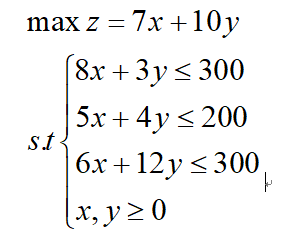在matlab中使用matlab标准的格式：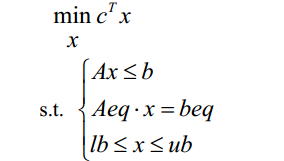若是目标函数是求解最大值的话，则取-C形式： 例如线性规划：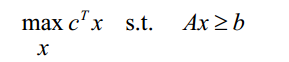的MATLAB标准型为：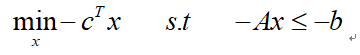二、linprog函数
在matlab中，linprog函数可以求解线性规划问题，用于寻找目标函数的最小值。 matlab中，规划模型的标注写法如下：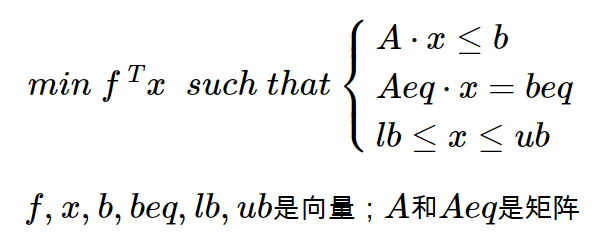（1）[x,fval]=linprog(f,A,b) 用于求解：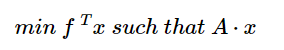（2）[x,fval]=linprog(f,A,b,Aeq,beq) 用于求解：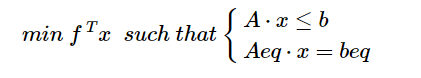如果没有等式存在，就用[]代替Aeq和beq。
（3）[x,fval]=linprog(f,A,b,Aeq,beq,lb,ub) 用于求解：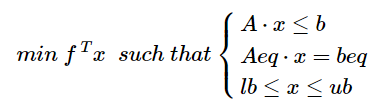返回的x为所求的结果，fval为最值。 linprog中fval都是求最小值，这个要记住。 A和b是不等式约束条件的参数。 Aeq和beq是等式约束条件的参数。 lb和ub为x取值的取值范围。
例如： a+b+c<30 c<15 b<10 函数：max f = 10a+20b+30c 因为linprog求的是最小值，我们将原式改为： f = -(10a+20b+30c) 这样我们有了函数f=[-10;-20;-30];，然后： 根据约束条件不等式，有： A = [1 1 1; 0 0 1; 0 1 0] ; b = [30;15;10] ; 但这样算出来的结果大家会发现是小数，也可能是负数。 因此我们加入a、b、c取值的上下限： lb = [0 0 0] ub = [30 30 30] 如果在计算中需要得到小数的结果，只要写成0.00或者30.00就可以了，最后带入函数计算就可以了。
三、intlinprog函数
intlinprog()是matlab中用于求解混合整数线性规划(Mixed-integer linear programming)的一个函数，用法基本和linprog差不多。 语法：
x=intlinprog(f,intcon,A,b,Aeq,beq,lb,ub)

与linprog相比，多了参数intcon,代表了整数决策变量所在的位置。 例如：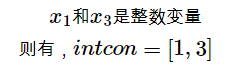四、Lingo软件
LINGO是用来求解线性和非线性优化问题的简易工具。 所有代码在 Lingo Model - Lingo 1中编写，写完后点击工具条上的红色的靶子运行。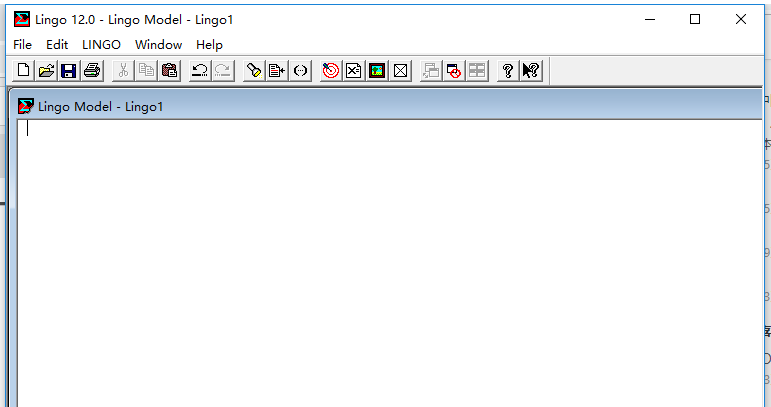例如：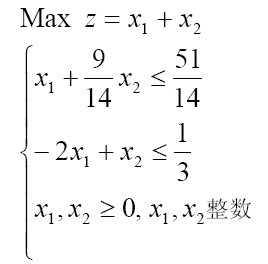代码：
max=x1+x2;
x1+9/14*x2<=51/14;
-2*x1+x2<=1/3;
@gin(x1);@gin(x2);
end

LINGO提供了大量的标准数学函数 @abs(x) 返回x 的绝对值 @sqrt() 开方 @sin(x) 返回x 的正弦值，x 采用弧度制 @cos(x) 返回x 的余弦值 @tan(x) 返回x 的正切值 @exp(x) 返回常数e 的x 次方 @log(x) 返回x 的自然对数 @lgm(x) 返回x 的gamma 函数的自然对数 @sign(x) 如果x<0 返回-1；否则，返回1 @floor(x) 返回x的整数部分。当x>=0 时，返回不超过x 的最大整数；当x<0 时，返回不低于x 的最大整数。 @smax(x1,x2,…,xn) 返回x1，x2，…，xn 中的最大值 @smin(x1,x2,…,xn) 返回x1，x2，…，xn 中的最小值
变量界定函数 变量界定函数实现对变量取值范围的附加限制，共4种 @bin(x) 限制x 为0 或1 — 用于0-1规划 @bnd(L,x,U) 限制L≤x≤U @free(x) 取消对变量x 的默认下界为0 的限制，即x 可以取任意实数 @gin(x) 限制x 为整数 在默认情况下，LINGO 规定变量是非负的，也就是说下界为0，上界为+∞。@free 取消 了默认的下界为0的限制，使变量也可以取负值。@bnd用于设定一个变量的上下界,它也可 以取消默认下界为0的约束。 注意： LINGO总是根据“MAX=”或“MIN=”寻找目标函数； 程序语句的顺序一般不重要，既可以随意调换； 程序运用函数时都是以@开头； 程序中的变量默认为非负数，想要改变变量类型必须有相应函数调整 程序中变量不区分大小写； 语句必须以分号结尾； 注释以!开始，且注释语句后面必须也有分号，注释默认注释到第一个分号处，意思是分号前面会全部被注释掉。
五、实例
根据外场改装合同要求，改装备件需于每季度末分别提供10、15、25、30。已知该专业厂各季度末的生产能力及每套产品的成本构成，如果专业厂生产出的备件当季不能交付，每套积压一季度需存储、维护等的费用0.15万元，建立一个数学模型，要求在完成合同的情况下，使该全年生产（包括存储、维护）费用最小。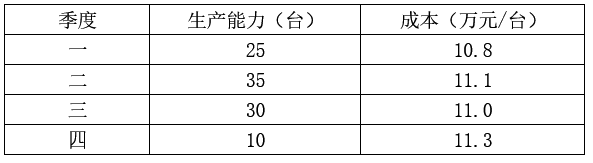解： 设x_ij为第i季度生产的用于第j季度交货产品的数量，则由题意得： x_11=10 x_12+x_22=15 x_13+x_23+x_33=25 x_14+x_24+x_34+x_44=30
又由生产能力的要求，有 x_44<=10 x_33+x_34<=30 x_22+x_23+x_24<=35 x_11+x_12+x_13+x_14<=25
再设c_ij表示第i季度生产的用于第j季度交货的每台产品的实际成本，其值如下表：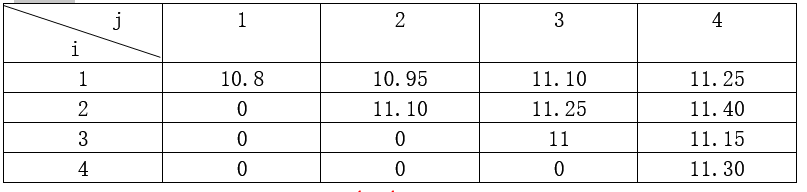目标函数为： min f = 10.8 * x11 + 10.95 * x12 + 11.10 * x13 + 11.25*x14 + 11.10 * x22 + 11.25 * x23 + 11.40 * x24 + 11 * x33 + 11.15 * x34 + 11.30 * x44;
MATLAB求解：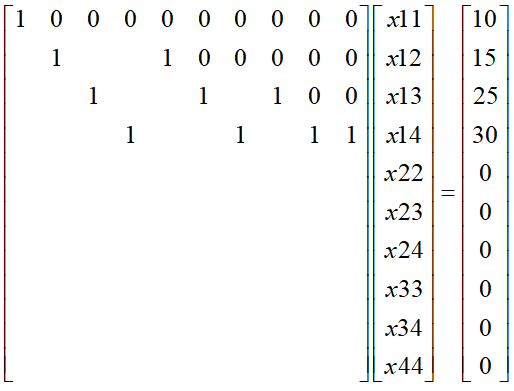上式中，Aeq为左边的矩阵，beq为等号右边的向量；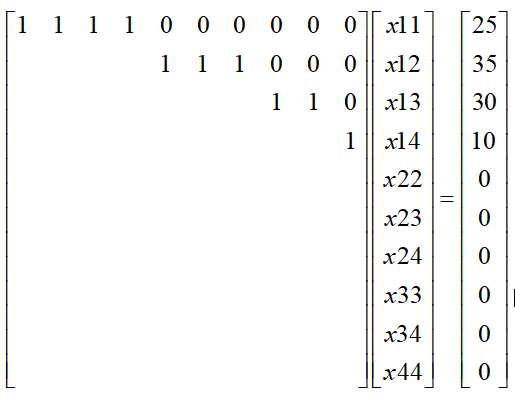上式中，A为左边的矩阵，b为等号右边的向量；
% 线性规划问题
% 目标函数：
% minF = 10.8 * x11 + 10.95 * x12 + 11.10 * x13 + 11.25 + x14 + ...
%    11.10 * x22 + 11.25 * x23 + 11.40 * x24 + ...
%    11 * x33 + 11.15 * x34 + ...
%    11.30 * x44;

minfun = [10.8 ,10.95 , 11.10 , 11.25 ,11.10 ,11.25,11.40 ,11,11.15,11.30]';
Aeq = zeros(10);
Aeq(1,1) = 1;
Aeq(2,2) = 1;  Aeq(2,5) = 1;
Aeq(3,3) = 1;  Aeq(3,6) = 1;  Aeq(3,8) = 1;
Aeq(4,4) = 1;  Aeq(4,7) = 1;  Aeq(4,9) = 1;  Aeq(4,10) = 1;
beq = [10,15,25,30,0,0,0,0,0,0]';
A = zeros(10);
A(1,1) = 1; A(1,2) = 1; A(1,3) = 1; A(1,4) = 1;
A(2,5) = 1; A(2,6) = 1; A(2,7) = 1;
A(3,8) = 1; A(3,9) = 1;
A(4,10) = 1;
b = [25,35,30,10,0,0,0,0,0,0]';
[x,y]=intlinprog(minfun,1:10,A,b,Aeq,beq,zeros(10,1)',[]);
disp('x的值为：');  x
disp('最小值为：');  y

运行结果： x的值为：
10
0
15
0
15
0
0
10
20
10

最小值为：
887
Lingo求解：
min=10.8 * x11 + 10.95 * x12 + 11.10 * x13 + 11.25*x14 + 11.10 * x22 + 11.25 * x23 + 11.40 * x24 + 11 * x33 + 11.15 * x34 + 11.30 * x44;
x11=10;
x12+x22=15;
x13+x23+x33=25;
x14+x24+x34+x44=30;
x11+x12+x13+x14<=25;
x22+x23+x24<=35;
x33+x34<=30;
x44<=10;
end

运行结果：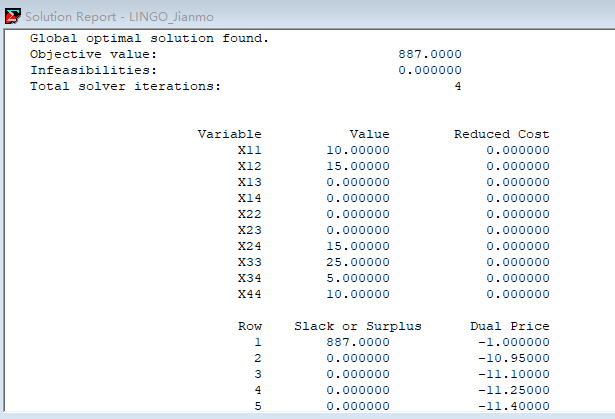最小值为：887
由此可见，MATLAB和lingo所求的最优解一致，但是每个变量值x并不一样，说明规划有多组解。 不足之处，请多指教！
展开全文matlab
• 附1：用LINGO求解线性规划的例子 一奶制品加工厂用牛奶生产A1、A2两种奶制品，1桶牛奶可以在设备甲上用12小时加工成3公斤A1，或者在设备乙上用8小时加工成4公斤A2。根据市场需求，生产的A1、A2能全部售出，且每...

附1：用LINGO求解线性规划的例子

一奶制品加工厂用牛奶生产A1、A2两种奶制品，1桶牛奶可以在设备甲上用12小时加工成3公斤A1，或者在设备乙上用8小时加工成4公斤A2。根据市场需求，生产的A1、A2能全部售出，且每公斤A1获利24元，每公斤A2获利16元。现在加工厂每天能得到50桶牛奶的供应，每天正式工人总的劳动时间为480小时，并且设备甲每天至多能加工100公斤A1，设备乙的加工能力没有限制。试为该厂制定一个生产计划，使每天获利最大，并进一步讨论以下3个附加问题：
1）若用35元可以购买到1桶牛奶，应否作这项投资？若投资，每天最多购买多少桶牛奶？
2）若可以聘用临时工人以增加劳动时间，付给临时工人的工资最多是每小时几元？
3）由于市场需求变化，每公斤A1的获利增加到30元，应否改变生产计划？
数学模型：设每天用x1桶牛奶生产A1 ，用x2桶牛奶生产A2
目标函数：设每天获利为z元。 x1桶牛奶可生产3x1公斤A1，获利24*3x1，x2桶牛奶可生产4*x2公斤A2，获利16*4x2，故z=72x1+64x2
约束条件：
原料供应：生产A1、A2的原料（牛奶）总量不超过每天的供应50桶，即
x1+x2≤50
劳动时间：生产A1、A2的总加工时间不超过每天正式工人总的劳动时间480小时，即
12x1+8x2≤480
设备能力：A1的产量不得超过设备甲每天的加工能力100小时，即
3x1≤100
非负约束：x1、x2均不能为负值，即x1≥0，x2≥0
综上所述可得
max z=72x1+64x2
s.t.
x1+x2≤50
12x1+8x2≤480
3x1≤100
x1≥0，x2≥0
显然，目标函数和约束条件都是线性的，这是一个线性规划（LP），求出的最优解将给出使净利润最大的生产计划，要讨论的问题需要考虑参数的变化对最优解和影响，一般称为敏感性（或灵敏度）分析。
LINGO求解线性规划
用LINGO求解线性规划时，首先在LINGO软件的模型窗口输入一个LP模型，模型以MAX或MIN开始，按线性规划问题的自然形式输入（见下面例子所示）。
以下解加工奶制品的生产计划问题：
由于LINGO中已假设所有的变量都是非负的，所以非负约束条件不必输入；LINGO也不区分变量中的大小写字符（实际上任何小写字符将被转换为大写字符）；约束条件中的“〈=”及“〉=”可用“〈”及“〉”代替。在LINGO模型窗口输入如下：
max=72*x1+64*x2;
x1+x2<=50;
12*x1+8*x2<=480;
3*x1<=100;
用鼠标单击菜单中的求解命令（Solve）就可以得到解答，结果窗口显示如下：
LP OPTIMUM FOUND AT STEP 2
OBJECTIVE FUNCTION VALUE
1)             3360.000
VARIABLE       VALUE          REDUCED COST
X1            20.000000                0.000000
X2            30.000000                0.000000
ROW    SLACK OR SURPLUS    DUAL PRICES
2)            0.000000              48.000000
3)            0.000000              2.000000
4)           40.000000              0.000000
NO. ITERATIONS=       2

计算结果表明：
“LP OPTIMUM FOUND AT STEP2”表示单纯形法在两次迭代（旋转）后得到最优解。
“OBJECTIVE FUNCTION VALUE 1) 3360.000”表示最优目标值为3360.000（LINGO中将目标函数自动看作第1行，从第二行开始才是真正的约束条件）。
“VALUE”给出最优解中各变量（VARIABLE）的值：x1=20.000000，x2=30.000000。
“REDUCED COST”的含义是（对MAX型问题）：基变量的REDUCED COST值为0，对于非基变量，相应的REDUCED COST值表示当非基变量增加一个单位时（其它非基变量保持不变）目标函数减少的量。本例中两个变量都是基变量。
“SLACK OR SURPLUS”给出松弛（或剩余）变量的值，表示约束是否取等式约束；第2、第3行松弛变量均为0，说明对于最优解而言，两个约束均取等式约束；第4行松弛变量为40.000000，说明对于最优解而言，这个约束取不等式约束。
“DUAL PRICES”给出约束的影子价格（也称为对偶价格）的值：第2、第3、第4行（约束）对应的影子价格分别48.000000，2.000000，0.000000。
“NO. ITERATIONS=       2”表示用单纯形法进行了两次迭代（旋转）。
灵敏度分析，则LINGO还会输出以下结果：
RANGES IN WHICH THE BASIS IS UNCHANGED:
OBJCOEFFICIENTRANGES
VARIABLE                        CURRENT                ALLOWABLE          ALLOWABLE
COEF           INCREASE         DECREASE
X1           72.000000         24.000000            8.000000
X2           64.000000          8.000000           16.000000
RIGHTHANDSIDERANGES
ROW        CURRENT        ALLOWABLE       ALLOWABLE
RHS            INCREASE         DECREASE
2            50.000000         10.000000          6.666667
3          480.000000         53.333332          80.000000
4          100.000000         INFINITY          40.000000
以上显示的是当前最优基（矩阵）保持不变的充分条件（RANGES IN WHICH THE BASIS IS UNCHANGED），包括目标函数中决策变量应的系数的变化范围（OBJ COEFFICIENT RANGES）和约束的右端项的变化范围（RIGHTHAND SIDE RANGES）两部分。
前一部分的输出行
X1           72.000000         24.000000            8.000000
表示决策变量X1当前在目标函数中对应的系数为72，允许增加24和减少8。也就是说，当该系数在区间[64，96]上变化时（假设其它条件均不变），当前最优基矩阵保持不变。对X2对应的输出行也可以类似地解释。由于此时约束没有任何改变，所以最优基矩阵保持不变意味着最优解不变（当然，由于目标函数中的系数发生变化，最优值还是会变的）。
后一部分的输出行
2            50.000000         10.000000          6.666667
表示约束2当前右端项为50，允许增加10和减少6.666667。也就是说，当该系数在区间[43.333333，60]上变化时（假设其它条件均不变），当前最优基矩阵保持不变。对约束3、约束4对应的输出行也可以类似地解释。由于此时约束已经改变，虽然最优基矩阵保持不变，最优解和最优值还是会变的。但是，由于最优基矩阵保持不变，所以前面的“DUAL PRICES”给出的约束的影子价格此时仍然是有效的。
用LINGO求解加工奶制品的生产计划，结果如下：
20桶牛奶生产A1, 30桶生产A2，利润3360元。
1）35元可买到1桶牛奶，要买吗？
由于原料的影子价格为48，35 <48, 应该买！
2）聘用临时工人付出的工资最多每小时几元？
由于工时的影子价格为2，聘用临时工人付出的工资最多每小时2元
3）A1获利增加到 30元/千克，应否改变生产计划
由于要使最优解保持不变，X1系数的允许变化范围为[64，96]。x1系数由24*3=72增加为30*3=90，在允许范围内。所以不改变生产计划。

转载于:https://www.cnblogs.com/flippedkiki/p/6942435.html
展开全文• matlab和Lingo求解线性方程-应用LINGO、MATLAB软件求解线性规划.pdf matlab和Lingo求解线性方程。matlab
• 数学建模学习中用到的lingo软件，进行线性规划的非常方便，lingo的强大不亚于matlab
• 经过整理，本问题的线性规划模型为 max=10*(x11+x21+x31)+8.2*(x12+x22+x32)+6.4*(x13+x23+x33)-2.5*(x11+x12+x13)-2*(x21+x22+x23)-1.7*(x31+x32+ x33); x11 >0.6*(x11+x21+ x31); x12 >0.15*(x12+x22+ x32); ...
• 第一步：输入目标条件和约束条件。每行以分号隔开。然后点击工具栏上的Solve按钮...需要在Lingo中一下配置才可以生成灵敏度分析报告：Lingo菜单》Options. General Solver选项卡》Dual Computations:Prices and Ran...
• LINGO软件求解“非线性规划”问题PDF讲义，适合初学者
• 解非线性规划 1二次型规划 标准型为 Min z-ITHx+ct s,t,Ax用 MATLAB软件求解,其输入格式如下: x-quadprog(h, C, a, b 2. x-quadprog(h, C, a, b, Aeg beg) 3. x=quadprog(H, C, A, b, Aeq, beq VLB, VUB) 4. x-...
• 目的 通过实现数学建模教材 P103 课后习题为例介绍如何使用 Lingo 软件设计线性规划求解模型。 习题 某银行经理计划用一笔资金进行有价证券的投资，可供购进的证券以及其信用等级、到期年限、收益如表1所示。按照...1024程序员节 数学建模
• Lingo求解线性规划问题，简明清晰，实例说明，适合初学者。
• 例：求解线性规划问题 max f = 3 x 1 − x 2 − x 3 \max f=3x_1-x_2-x_3 s . t . ⎧ ⎩ ⎨ ⎪ ⎪ ⎪ ⎪ ⎪ ⎪ ⎪ ⎪ x 1 − x 2 + x 3 ≤ 11 − 4 x 1 + x 2 + 2 x 3 ≥ 3 2 x 1 − x 3 = − 1 x i ≥ 0 , ...matlab
• Lingo版本： 某工厂明年根据合同，每个季度末向销售公司提供产品，有关信息如下表。若当季生产的产品过多，季末有积余，则一个季度每积压一吨产品需支付存贮费O.2万元。现该厂考虑明年的最佳生产方案，使该厂...
• 数学建模学习笔记（2.3）lingo软件求解线性规划问题 lingo软件的优势在于体积小，专注于解决优化问题 且编程语言通俗易懂，没有门槛 对于刚刚接触数学建模同学比较友善 当然对于已经参与建模很久的老司机来说，...
• 于是，我们得本问题的线性规划模型：  minf=3x12+x13+3x22+x23+x24+4x25+2x26+4x2+2x3+2x4+5x5  s．t． 2x11+4x21+2x22+2x23+x24+x2+x4- x5=10000  x12+x22+2x24+x25+x3- x4=30000  x13+x23+x25+2x26- ...
• 线性规划是运筹学中研究较早、发展较快、应用广泛、方法较成熟的一个重要分支，它是辅助人们进行科学管理的一种数学方法。 在经济管理、交通运输、工农业生产等经济活动中，提高经济效果是人们不可缺少的要求，而...
• 文章目录一、算法介绍二、适用问题三、算法总结四、应用场景举例（lingo求解）五、LINGO代码六、实际案例七、论文案例片段（待完善） 线性规划模型主要针对数学建模问题中的一些小的子问题进行求解，如果想直接使用...算法 人工智能 机器学习 数学建模
• ## LINGO求解规划问题代码

千次阅读 多人点赞 2020-08-21 23:10:08
文章目录线性规划标量线性规划矩阵线性规划线性规划无特殊限制整数规划01规划 写在开头： LINGO默认所有变量是非负的。 LINGO中没有严格的<和>，<代表<=，>代表>=。 LINGO不区分变量的大小写。...
• 求解线性规划LINGO程序，约束180个左右，变量100个左右！
•数学建模
• Lingo程序： max=1.7*x42+1.45*x23+1.3*x14+1.1* x55; x11 +x21+x31+x51=300000 ; x12 +x22+x42+x52=1.1* x51; x13 +x23+x53=1.1*x52+1.3* x11; x14 +x54=1.1*x53+1.3*x12+1.45* x21; x55 =1.1*x54+1.3*x13+1.45...
• ## Lingo —— 求解规划问题

万次阅读 多人点赞 2018-08-23 18:05:29
线性规划 （1）定义：线性规划问题是在一组线性约束条件的限制下，求一线性目标函数的最优解的问题。 （2）举例： model: min=@abs(x1)+2*@abs(x2)+3*@abs(x3)+4*@abs(x4);! 目标函数; x1-x2-x3+x4=0; x1-...
• Lindo 用于求解线性规划和二次规划问题,Lingo 除了具有 Lindo 的全部功能外,还可以用于求解非线性规划问题,也可以用于一些线性和非线性方程（组）的求解,等等。Lindo 和 Lingo 软件的最大特色在于可以允许优化模型中...
• ## 数模2 Lingo解决线性规划问题

万次阅读 多人点赞 2018-09-07 10:06:46
LP模型在Lingo中的一个典型输入方式：  1.以“MODEL:”开。  2.集合定义部分从(“SETS:”到”ENDSETS”)定义集合及其属性。  3.给出优化目标和约束。  4.数据定义部分从(“DATA:”到”ENDDATA”)。  5.以”END...
• 解决一个线性规划问题的lingo程序。 片段： model: sets: r/1..6/:; c/1..5/:d; z/1..30/:; link(r,c):m,n,p; endsets
• 本文讲解了规划问题的几个要点，并附有案例及代码传送门。算法 数学建模...